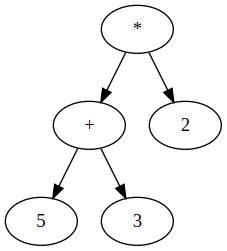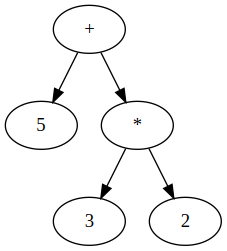# Optimizing Parser Performance

Tue May 17 2022 by Mark Sujew

When designing and implementing DSLs, performance is often an afterthought. However, once your DSL moves into production and users start to build large projects with it, they can quickly hit a performance roadblock. In this blog post we will explore different options to improve parser performance for top-down parsers, which are commonly used in DSL projects.

## Operator Precedence Parsing

If your DSL contains any sort of mathematical expressions, you probably also had to deal somehow with operator precedence. For this, you would usually set up your parser as an Operator-Precedence Parser. While an operator-precedence parser automatically handles any kind of precedence without post-processing, it can often lead to increased parsing time, due to the large amount of rule calls performed by the parser. Let's take a look at a practical example from an Xtext grammar:

``````Expression: Addition;

Multiplication ({BinaryExpression.left=current} operator=('+' | '-') right=Multiplication)*;

Multiplication returns Expression:
Power ({BinaryExpression.left=current} operator=('*' | '/') right=Power)*;

Power returns Expression:
Primary ({BinaryExpression.left=current} operator=('**') right=Primary)*;

Primary returns Expression:
'(' Expression ')' | {NumberLiteral} value=NUMBER;
``````

In order to parse a single number, the parser has to enter through the `Expression` rule and descend through the `Addition`, `Multiplication` and `Power` rules until it reaches the `value=NUMBER` assignment of the `Primary` rule. This results in 4 rule calls and 4 lookahead calls. Both of these numbers increase linearly for each additional level of precedence. Complex expression languages are likely to have 7 or more levels of precedence.

In a first step to increase parsing performance, we can completely remove these steps, and parse every expression in two steps:

``````Expression: Primary ({BinaryExpression.left=current} operator=('+' | '-' | '*' | '/' | '**') right=Primary)*;

Primary returns Expression:
'(' Expression ')' | {NumberLiteral} value=NUMBER;
``````

While this improves performance, we've now lost our operator precedence. However, we are able to reinstate operator precedence just before running our code generator or interpreter. We can do this by rebalancing the expression tree. An expression such as `5 + 3 * 2` will look as follows in our parse tree:How our expression tree looks likeHow it needs to look like

Now, to turn the expression tree on the left to the expression tree on the right, we need to rotate the tree to the right at specific nodes. In particular, we rotate every time we encounter an operator with a higher precedence than its parent node operator. See the following Java pseudo-code snippet on how this could be performed:

``````BinaryExpression sortByPrecedence(BinaryExpression binaryExpression) {
BinaryExpression expression = binaryExpression;
while (expression.left instanceof BinaryExpression && !inParenthesis(expression.left)) {
BinaryExpression left = expression.left;
if (getPrecedence(expression.op) > getPrecedence(left.op)) {
// Rotate the expression tree if the precedence is higher
expression = rotateRight(expression);
} else {
expression = left;
}
}
// Find the top most binary expression and return it
while (expression.container instanceof BinaryExpression) {
expression = expression.container;
}
return expression
}

BinaryExpression rotateRight(binaryExpression: BinaryExpression) {
BinaryExpression leftChild = (BinaryExpression)binaryExpression.left;
binaryExpression.left = leftChild.right;

// Keep the container chain alive by setting the left hand side of the old container
Expression oldParent = (Expression)binaryExpression.container
if (oldParent instanceof BinaryExpression) {
oldParent.left = leftChild;
}

leftChild.right = binaryExpression;
return leftChild
}
``````

In the end, we're left with an expression tree which looks like it was parsed using an operator-precedence parser, while still enjoying the performance benefits of a single-layer expression parser.

How much speed do we actually gain from this? We did some small benchmarks using Chevrotain and were seeing 25% performance improvements for a pure expression-based language when parsing numbers. These benefits diminish quickly when parsing complex expressions, but it's an easy performance gain when you can expect that a lot of parsed "expressions" are actually just numbers.

Top-down parsers usually require to look into the input token stream to determine which alternative to parse. For example for the rule `A: ('a' 'c' | 'b' 'c')`, the parser needs to look at the first incoming token and see whether it matches `a` or `b`. Afterwards, it knows which alternative to parse and can go on parsing as usual. Most alternatives however aren't that simple. When looking at fairly complex programming languages like Java, seemingly simple tasks such as parsing a field or method signature are quite complex from a lookahead perspective:

``````ClassBody: (elements+=Field | elements+=Method | elements+=Constructor)*;

Field: modifiers+=Modifiers* type=[Type] name=ID ...;

Method: modifiers+=Modifiers* type=[Type] name=ID '(' ...;

Constructor: modifiers+=Modifiers* name=ID ...;
``````

One can quickly see where the problem begins - most top-down parsers will have trouble deciding between the `Field`, `Method` and `Constructor` rules, since they start similarly. Even worse, their common start can contain an arbitrary amount of accessibility modifiers. None of the commonly used LL(k) parsers are able to deal with this, and even LL(*) parsers (such as ANTLR4) need some time to identify which grammar rule to parse. However, by extracting the common prefix, we can minimize the necessary lookahead:

``````ClassBody: elements+=ClassBodyElement*;

ClassBodyElement: modifiers+=Modifiers* element=Member;

Member: Field | Method | Constructor;

Field: type=[Type] name=ID ...;

Method: type=[Type] name=ID '(' ...;

Constructor: name=ID ...;
``````

In our benchmarks, reducing LL(k) decisions to LL(1) improves performance of each specific decision by up to 20%.

## Production ordering

Most top-down parsers are influenced by the order in which productions appear. For example, a lot of them will have trouble with the parser rule `X: ('a' | 'a' 'b')`, since they usually search for the first matching alternative, not the longest. Consequently, the second alternative is never parsed, since the first has precedence over it.

However, we don't only have to think about alternative ordering when concerned with correctness. A simpler example `A: ('a'| 'b')` might be subject to reordering if we know that `b` is more likely to appear than `a`. Putting `'b'` first allows us to skip the `'a'` alternative, making the parser faster under the assumption that `b` appears more often.

Another case where ordering matters is related to the lexing phase. Lexers often also look for the first matching token, instead of the longest. In the same vein as earlier, we can reorder our terminals in order of appearance. Statistically speaking, the `whitespace` token is the likeliest to appear in your DSL, so it should appear first, then any keywords, and then longer tokens, such as IDs, strings or numbers.

Keep in mind that some parsing libraries have their own quirks and special behavior, which puts this advice in question. In ANTLR4 for example, production and token order is completely irrelevant, while others such as Chevrotain or PEGjs rely on specific ordering.

Under optimal conditions we've seen performance improvements for optimized alternatives of approximately 10%. This assumes that the majority (> 90%) of parsed decisions resolve to a specific alternative which we placed as the first alternative.

## Conclusion

In this article we showcased 3 ways of improving parsing performance. These are general tips that should be applicable to most top-down parsing libraries. If you're curious about ANTLR specific optimizations, the folks over at Strumenta did a great writeup on ANTLR4 optimizations.